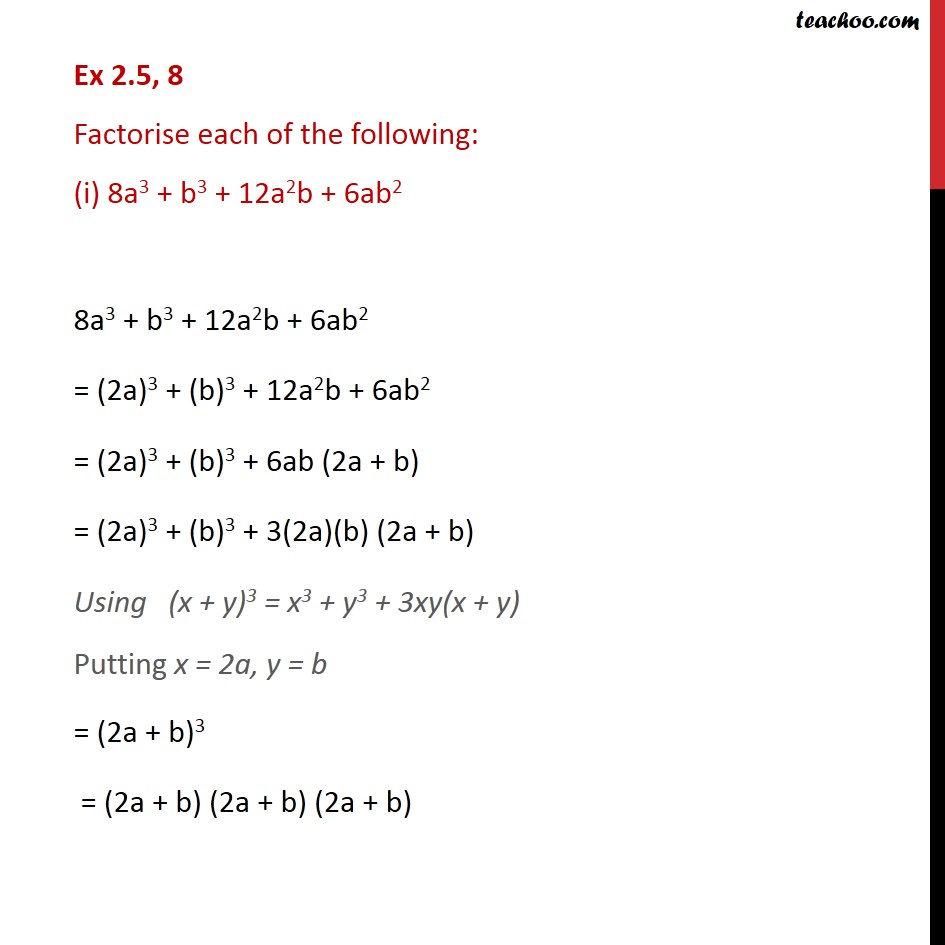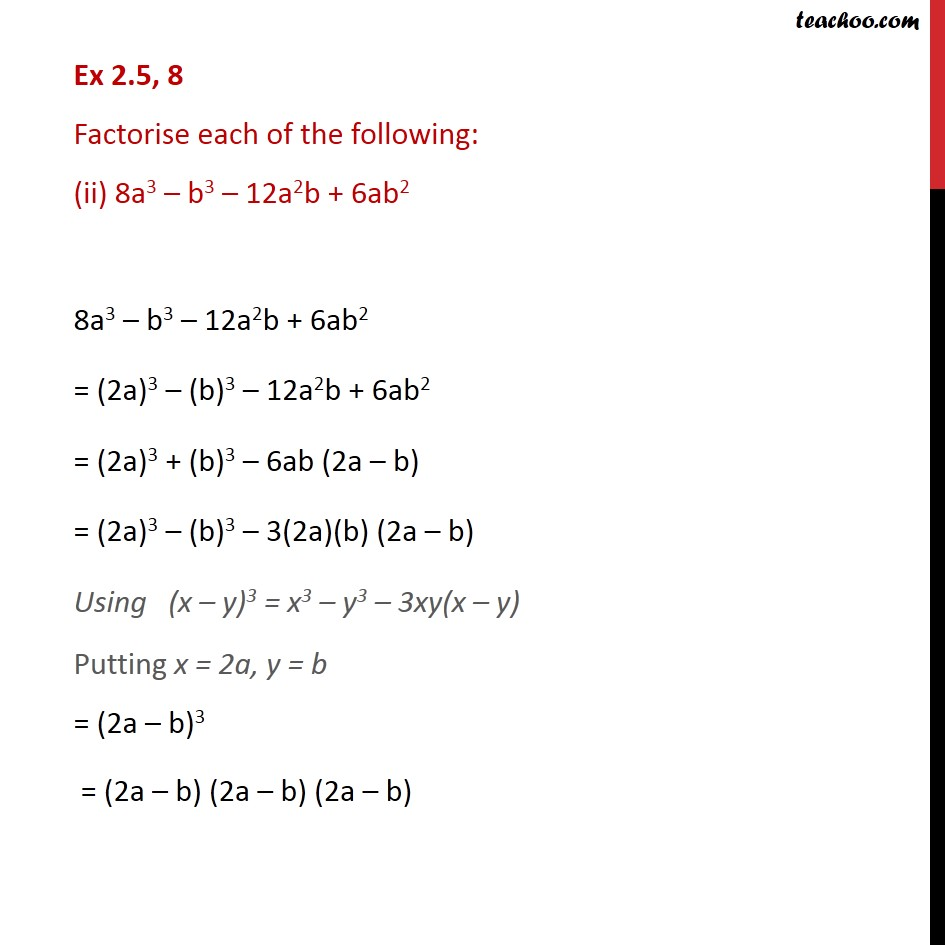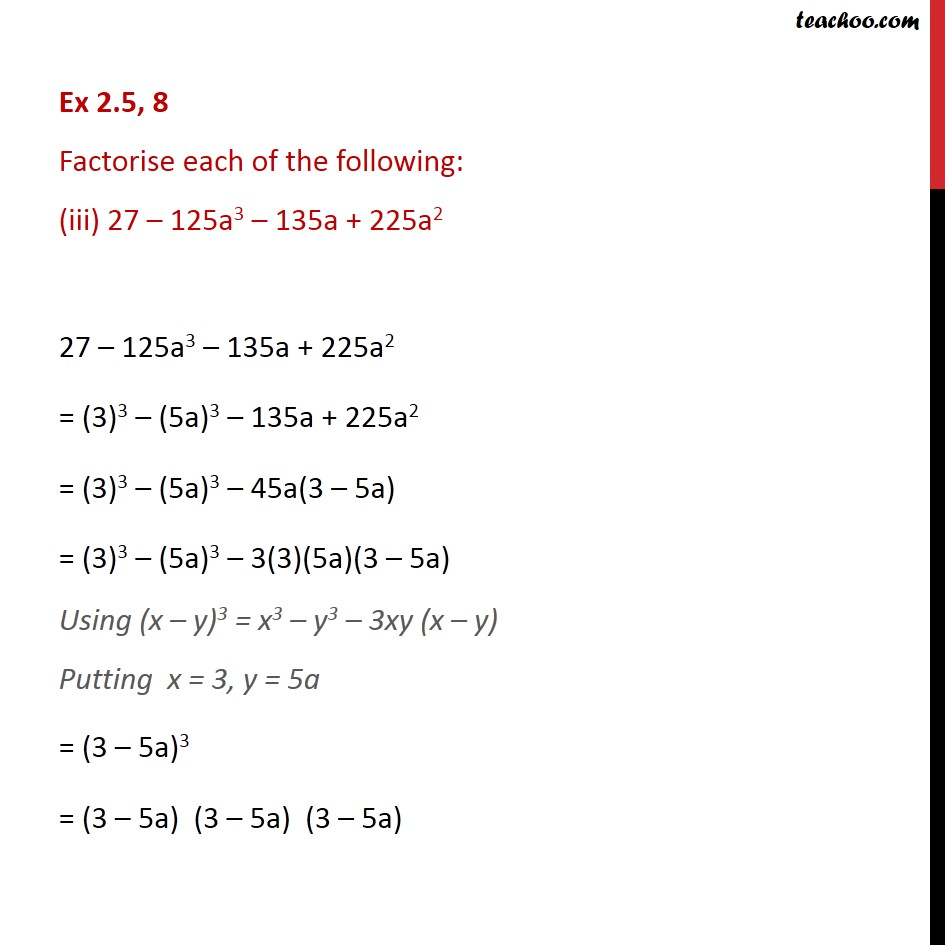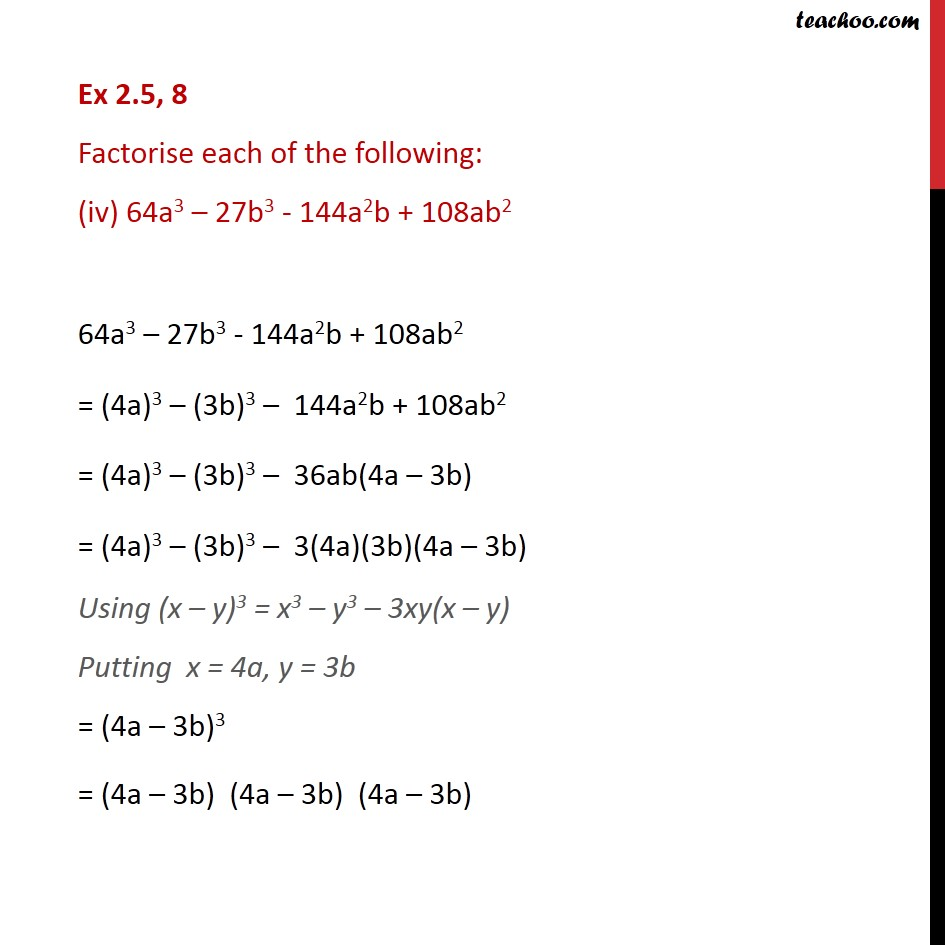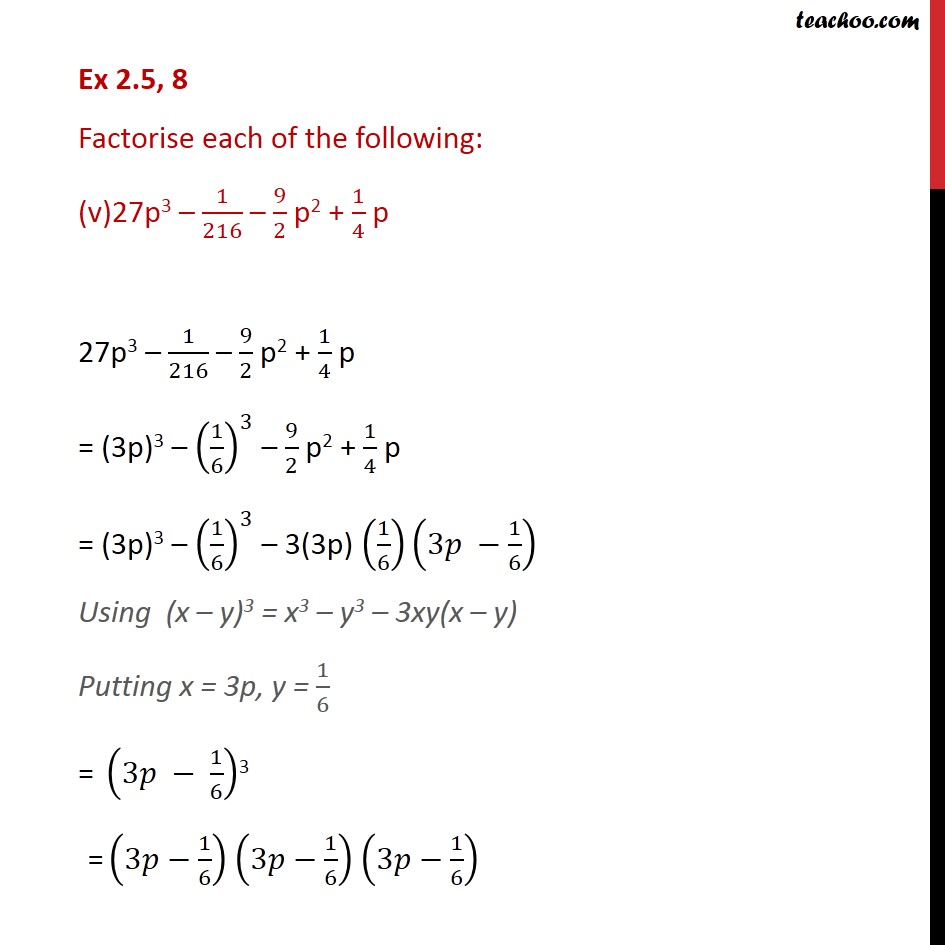1. Chapter 2 Class 9 Polynomials
2. Serial order wise
3. Ex 2.5

Transcript

Ex 2.5, 8 Factorise each of the following: (i) 8a3 + b3 + 12a2b + 6ab2 8a3 + b3 + 12a2b + 6ab2 = (2a)3 + (b)3 + 12a2b + 6ab2 = (2a)3 + (b)3 + 6ab (2a + b) = (2a)3 + (b)3 + 3(2a)(b) (2a + b) Using (x + y)3 = x3 + y3 + 3xy(x + y) Putting x = 2a, y = b = (2a + b)3 = (2a + b) (2a + b) (2a + b) Ex 2.5, 8 Factorise each of the following: (ii) 8a3 – b3 – 12a2b + 6ab2 8a3 – b3 – 12a2b + 6ab2 = (2a)3 – (b)3 – 12a2b + 6ab2 = (2a)3 + (b)3 – 6ab (2a – b) = (2a)3 – (b)3 – 3(2a)(b) (2a – b) Using (x – y)3 = x3 – y3 – 3xy(x – y) Putting x = 2a, y = b = (2a – b)3 = (2a – b) (2a – b) (2a – b) Ex 2.5, 8 Factorise each of the following: (iii) 27 – 125a3 – 135a + 225a2 27 – 125a3 – 135a + 225a2 = (3)3 – (5a)3 – 135a + 225a2 = (3)3 – (5a)3 – 45a(3 – 5a) = (3)3 – (5a)3 – 3(3)(5a)(3 – 5a) Using (x – y)3 = x3 – y3 – 3xy (x – y) Putting x = 3, y = 5a = (3 – 5a)3 = (3 – 5a) (3 – 5a) (3 – 5a) Ex 2.5, 8 Factorise each of the following: (iv) 64a3 – 27b3 - 144a2b + 108ab2 64a3 – 27b3 - 144a2b + 108ab2 = (4a)3 – (3b)3 – 144a2b + 108ab2 = (4a)3 – (3b)3 – 36ab(4a – 3b) = (4a)3 – (3b)3 – 3(4a)(3b)(4a – 3b) Using (x – y)3 = x3 – y3 – 3xy(x – y) Putting x = 4a, y = 3b = (4a – 3b)3 = (4a – 3b) (4a – 3b) (4a – 3b) Ex 2.5, 8 Factorise each of the following: (v)27p3 – 1/216 – 9/2 p2 + 1/4 p 27p3 – 1/216 – 9/2 p2 + 1/4 p = (3p)3 – (1/6)^3 – 9/2 p2 + 1/4 p = (3p)3 – (1/6)^3 – 3(3p) (1/6)(3𝑝 −1/6) Using (x – y)3 = x3 – y3 – 3xy(x – y) Putting x = 3p, y = 1/6 = (3𝑝 − 1/6)3 = (3𝑝−1/6) (3𝑝−1/6) (3𝑝−1/6)

Ex 2.5

Chapter 2 Class 9 Polynomials
Serial order wise

About the AuthorDavneet Singh
Davneet Singh is a graduate from Indian Institute of Technology, Kanpur. He has been teaching from the past 10 years. He provides courses for Maths and Science at Teachoo.